Question

# Ethyl alcohol has a boiling point of 78.0 degrees Celsius, a freezing point of -114 degrees...

Ethyl alcohol has a boiling point of 78.0 degrees Celsius, a freezing point of -114 degrees Celsius, a latent heat of vaporization of 879 kJ/K, a latent heat of fusion of 109 kJ/K, and a specific heat of 2.43 kJ/kg.K. How much energy must be removed from 0.510 kg of ethyl alcohol that is initially a gas at 78.0 degrees Celsius so that it becomes a solid at -114 degrees Celsius?

here,

the mass of ethyl alcohol , m = 0.51 kg

initial temperature , Ti = 78 degree C

the final temperature , Tf = - 114 degree C

the heat energy required , Q = m * ( Lv + Ce * ( Ti - Tf) + Lf)

Q = 0.51 * ( 879 + 2.43 * ( 78 - (-114) + 109 ) KJ

Q = 742 KJ

#### Earn Coins

Coins can be redeemed for fabulous gifts.

Similar Homework Help Questions
• ### Ethyl alcohol has a boiling point of 78.0 ˚C, a freezing point of -114 ˚C, a...

Ethyl alcohol has a boiling point of 78.0 ˚C, a freezing point of -114 ˚C, a heat of vaporization of 879 kJ/kg, a heat of fusion of 109 kJ/kg, and a specific heat of 2.43 kJ/kg·K. How much energy must be removed from 0.689 kg of ethyl alcohol that is initially a gas at 78.0 ˚C so that it becomes a solid at -114 ˚C?

• ### Ethyl alcohol has a boiling point of 78.0 ˚C, a freezing point of -114 ˚C, a...

Ethyl alcohol has a boiling point of 78.0 ˚C, a freezing point of -114 ˚C, a heat of vaporization of 879 kJ/kg, a heat of fusion of 109 kJ/kg, and a specific heat of 2.43 kJ/kg·K. How much energy must be removed from 0.683 kg of ethyl alcohol that is initially a gas at 78.0 ˚C so that it becomes a solid at -114 ˚C?

• ### Ethyl alcohol has a boiling point of 78.0 degree C, a freezing point of -114 degree...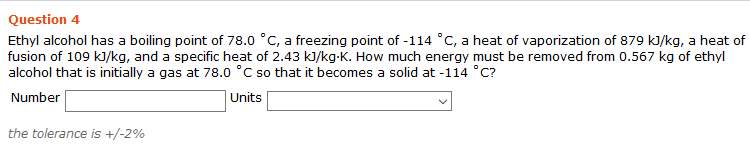Ethyl alcohol has a boiling point of 78.0 degree C, a freezing point of -114 degree C, a heat of vaporization of 879 kJ/kg, a heat of fusion of 109 kJ/kg, and a specific heat of 2.43 kJ/kg-K. How much energy must be removed from 0.567 kg of ethyl alcohol that is initially a gas at 78.0 degree C so that it becomes a solid at -114 degree C?

• ### Question 5 Ethyl alcohol has a boiling point of 78.0 °C, a freezing point of -114...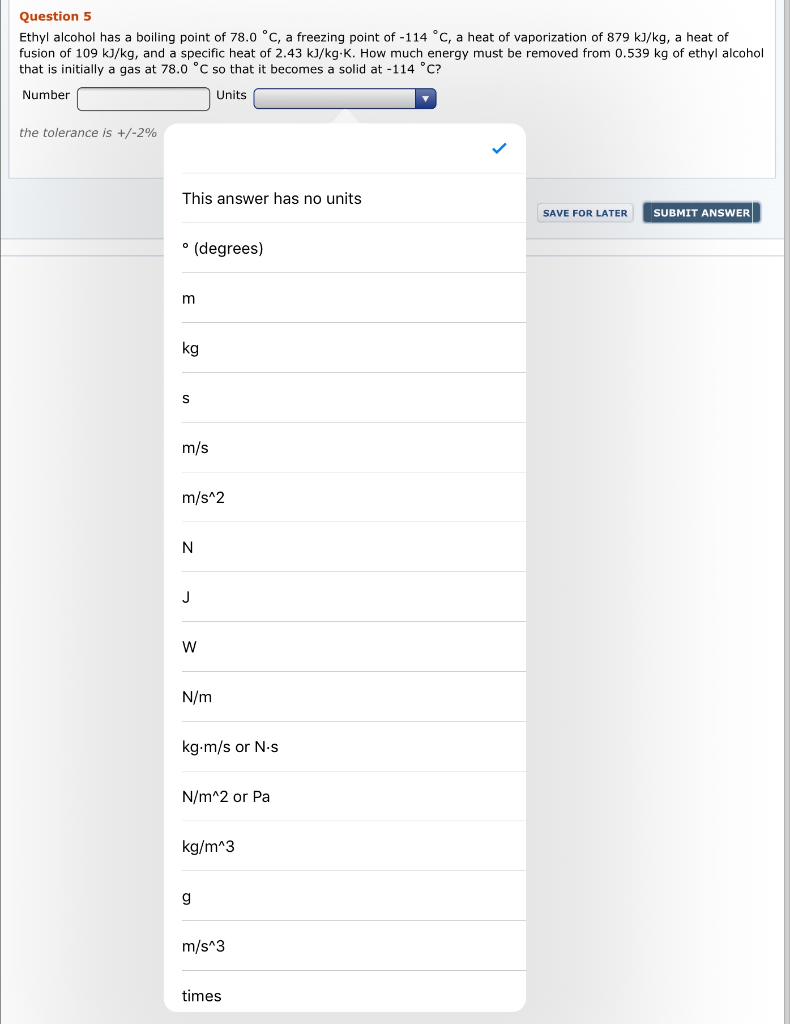Question 5 Ethyl alcohol has a boiling point of 78.0 °C, a freezing point of -114 °c, a heat of vaporization of 879 kJ/kg, a heat of fusion of 109 kJ/kg, and a specific heat of 2.43 kJ/kg-K. How much energy must be removed from 0.539 kg of ethyl alcohol that is initially a gas at 78.0 °C so that it becomes a solid at -114 °C? Number Units the tolerance is +/-2% This answer has no units SAVE FOR...

• ### Chapter 18, Problem 039 Ethyl alcohol has a boiling point of 78.0 °C, a freezing point...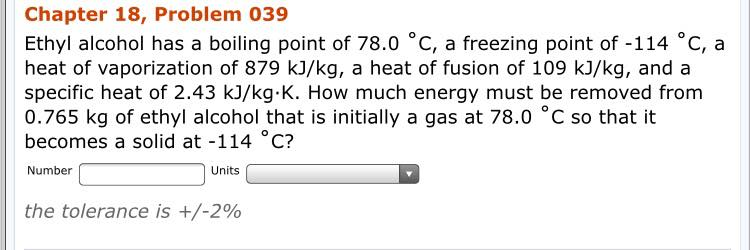Chapter 18, Problem 039 Ethyl alcohol has a boiling point of 78.0 °C, a freezing point of -114 °C, a heat of vaporization of 879 kJ/kg, a heat of fusion of 109 kJ/kg, and a specific heat of 2.43 kJ/kg K. How much energy must be removed from 0.765 kg of ethyl alcohol that is initially a gas at 78.0 °C so that it becomes a solid at -114 C? Number Units the tolerance is +/-2%

• ### Need help on those two problems! Question 4 Incorrect. Ethyl alcohol has a bolling point of...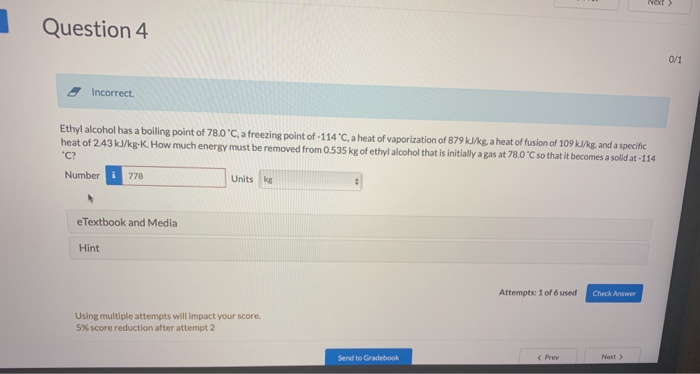Need help on those two problems! Question 4 Incorrect. Ethyl alcohol has a bolling point of 780°C a freezing point of-1 14 С a heat of vaporization of 879 kJkg a heat of fusion of 109 kle and a specific C? Number778 heat of 243kJkgK. Howmuch enersy must be removed from Os535 kg of ethylalcoho that sinitialy gas at 780 C so that i becomes a sold a 114 Units kg eTextbook and Media Hint Attempts: 1 of 6 used...

• ### stored at 12C. The specific heat of fish above freezing point is 680 kg of fish...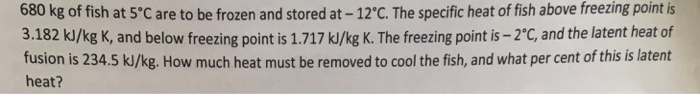stored at 12C. The specific heat of fish above freezing point is 680 kg of fish at 5'C are to be frozen and 3.182 kJ/kg K, and below freezing point is 1.717 kJ/kg K. The freezing point is -2'C, and the latent heat of fusion is 234.5 k/kg. How much heat must be removed to cool the fish, and what per cent of this is latent heat?

• ### Acetone has a melting point of -95degree Celsius and a boiling point of 56 degrees Celsius....

Acetone has a melting point of -95degree Celsius and a boiling point of 56 degrees Celsius. Which phase (solid, liquid, or gas) is present at the following temperature?1. Inside this room(25 degree Celsius) . 2 . Dark side of the moon ( - 153 degrees Celsius) . 3. Outside on a very cold winter morning (-5 degrees Celsius). 4. Light side of the moon (123 degrees Celsius) . 5 . Very hot summer day (38 degrees Celsius)

• ### Liquid ammonia (boiling point = -33.4 degrees Celsius) can be usedas a refrigerant and heat transfer...

Liquid ammonia (boiling point = -33.4 degrees Celsius) can be usedas a refrigerant and heat transfer fluid. how much energy is neededto heat 25.0 g of NH3 (liquid) from -65.0 degreesCelsius to -12.0 degrees Celsius ? specific heat capacity, NH3 (liquid)   4.7 J/ (g x k) specific heat capacity, NH3 (gas)      2.2J/ (g x k) heat ofvaporization                  23.5 kJ/mol molarmass,                    17.0 g/mol correct answer: 39 kJ please show me step by step how to solve this problem, i'm notgetting the correct answer...

• ### An aqueous solution of a nonvolatile solute has a boiling point of 102.45 degrees Celsius. Knowing...

An aqueous solution of a nonvolatile solute has a boiling point of 102.45 degrees Celsius. Knowing that for water, Kfp = 1.86 degrees Celsius m^-1 and Kbp= 0.51 degrees Celsius m^-1. What is the molality of the solution? and what is the freezing point of the solution? Please show work.